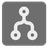Merge Intervals
Simple
English

You are given a sequence of intervals, as tuples of ints where the tuples are sorted by their first element in ascending order.
It is your task to minimize the number of intervals by merging those that intersect or by removing intervals fitting into another one.

Since the range of values for the intervals is restricted to integers, you must also merge those intervals between which no value can be found.

An example:
Let's say you've got these 5 intervals: 1..6, 3..5, 7..10, 9..12 and 14..16.

1. 1..6 and 3..5
3..5 fits into 1..6, so 3..5 must disappear.
2. 1..6 and 7..10
Even though the intervals do not intersect, there can not be a value of type int between them, so they have to be merged to the new interval 1..10.
3. 1..10 and 9..12
These intervals do intersect, because 9 < 10, so they have to be merged to the new interval 1..12.
4. 1..12 and 14..16
These two intervals do not intersect, so they must not be merged.
So the intervals to be returned are:
1..12 and 14..16

Input: A sequence of intervals as a list of tuples of 2 ints, sorted by their first element.

Output: The merged intervals as a list of tuples of 2 ints, sorted by their first element.

Examples:

```merge_intervals([(1, 4), (2, 6), (8, 10), (12, 19)]) == [(1, 6), (8, 10), (12, 19)]
merge_intervals([(1, 12), (2, 3), (4, 7)]) == [(1, 12)]
merge_intervals([(1, 5), (6, 10), (10, 15), (17, 20)]) == [(1, 15), (17, 20)]```

Precondition:

• intervals == sorted(intervals, key=lambda x: x) # sorted by 1st element of the tuples
• interval <= interval